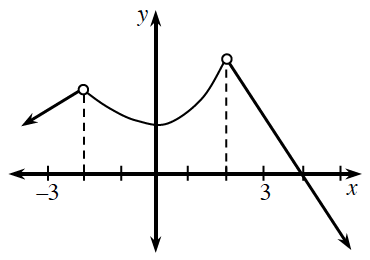### Home > PC3 > Chapter 7 > Lesson 7.2.2 > Problem7-87

7-87.The graph of $y=g(x)$ is shown at right. Does $\lim\limits _ { x \rightarrow b } g ( x )$ exist for:

Limits do not exist when there are vertical asymptotes or "jumps" in the curve.

1. $b=-3$

2. $b=-2$

3. $b=2$

4. All values of $b$ in the interval $-3

5. Is the function continuous at $x=2$? Explain.

For a function to be continuous at a given point, the limit must exist and the function must be defined at that point.